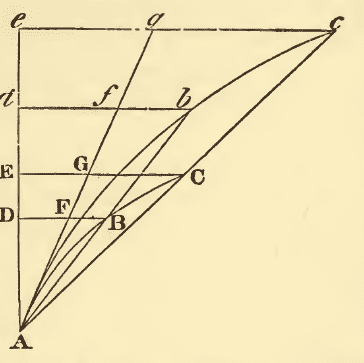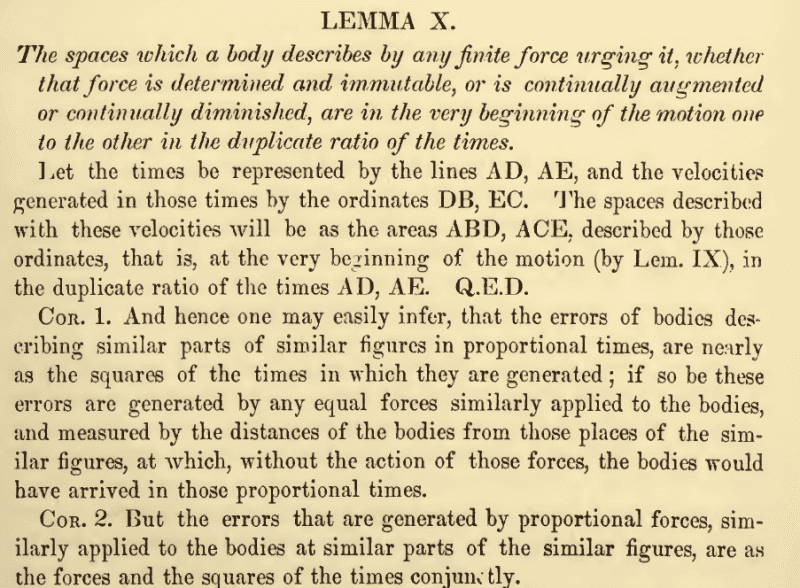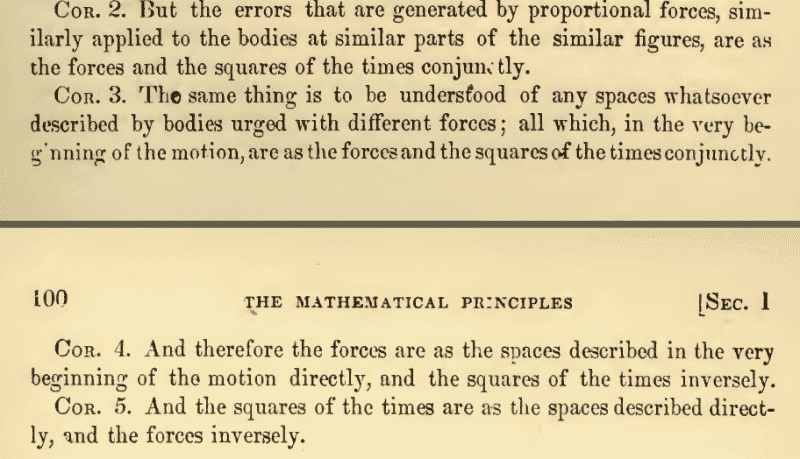# Newton's Principia Problem

• Solomon Chang
In summary, the conversation is about understanding Newton's Principia and its notation. The participants discuss the difficulty in understanding Lemma 10 and ask for help in interpreting it. They also mention the possibility of understanding the Principia in a more numerical way. One person shares their understanding of the notation in modern terms. They also discuss the translation of the Principia and its difficulty in reading.

#### Solomon ChangFurthermore, is there any way of understanding the Principia in a more numerical ways rather than words like this? Just asking.
Again, thank you very much.

S. Chandrasekhar Newton's Principia for the Common Reader Chapter 3, Section 15, pp 50-52 in modern integral notation.

I think that in modern terms, this is $s = \frac{1}{2} a t^2$. So $\frac{s_1}{s_2} = (\frac{t_1}{t_2})^2$. This is constant acceleration from rest. Since it's at the very beginning off the motion, the force won't have had time to change?

Last edited:
It would interesting to know whether Newton is representing a graph of a function of position vs time with time on the y-axis or whether he is thinking of the curves as trajectories of an object in 2-D space.

Wao, thank you everyone, this helps much

The English translation (of Newton's original Latin) that you are reading dates from around 1730 and can be difficult to read, even for native English speakers. This book contains a new translation from the late 1990s, along with an extensive "guide to the Principia" which is as long as the Principia itself (i.e. half the book)!

https://www.amazon.com/dp/0520088174/?tag=pfamazon01-20

Last edited by a moderator:

## 1. What is Newton's Principia Problem?

Newton's Principia Problem refers to the challenge that Sir Isaac Newton faced in developing his famous theory of gravity, which he presented in his book "Philosophiae Naturalis Principia Mathematica".

## 2. What was the main idea behind Newton's Principia Problem?

The main idea behind Newton's Principia Problem was to explain the motion of objects in the universe, specifically the motion of planets around the sun, using a single set of mathematical laws.

## 3. What were the key principles introduced in Newton's Principia Problem?

The key principles introduced in Newton's Principia Problem include the law of inertia, the law of acceleration, and the law of action and reaction. These principles formed the basis of Newton's theory of gravity.

## 4. Why is Newton's Principia Problem considered significant?

Newton's Principia Problem is considered significant because it provided a new understanding of the physical world and revolutionized the fields of mathematics and physics. It also paved the way for future developments in these fields, leading to groundbreaking discoveries and advancements.

## 5. How did Newton's Principia Problem impact science and society?

Newton's Principia Problem had a significant impact on both science and society. It laid the foundation for modern physics and influenced the development of many other scientific fields. It also led to advancements in technology, such as space exploration and satellite navigation, which have greatly benefited society.